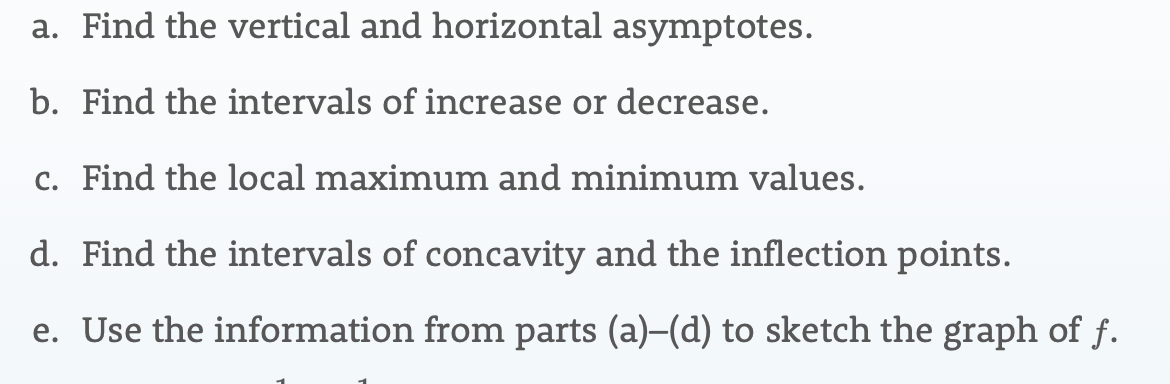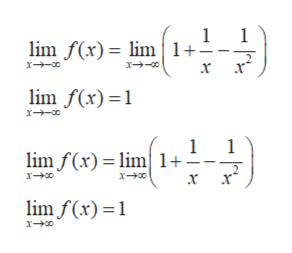# a. Find the vertical and horizontal asymptotes.b. Find the intervals of increase or decrease.c. Find the local maximum and minimum values.d. Find the intervals of concavity and the inflection points.e. Use the information from parts (a)-(d) to sketch the graph of f.

Question
117 views

f(x) = 1 + (1/x) - (1/x^2)help_outlineImage Transcriptionclosea. Find the vertical and horizontal asymptotes. b. Find the intervals of increase or decrease. c. Find the local maximum and minimum values. d. Find the intervals of concavity and the inflection points. e. Use the information from parts (a)-(d) to sketch the graph of f. fullscreen
check_circle

Step 1

The given function is

Step 2

To find:

• The vertical and horizontal asymptotes of the function.
• The interval of concavity and the inflection point.
• Sketch the graph of the function using information from sub-parts (a) and (d).
Step 3
• The function will have vertical asymptotes at those points at which denominator of the function is equal to 0.

Therefore, substitute denominator of (1) equal to 0 gives, x2 = 0, i.e. x = 0.

Hence x = 0 is the vertical asymptote of t...help_outlineImage Transcriptionclose1 1 lim f(x)= lim 1+ x lim f(x) 1 1 lim f(x) lim 1+- lim f(x) 1 fullscreen

### Want to see the full answer?

See Solution

#### Want to see this answer and more?

Solutions are written by subject experts who are available 24/7. Questions are typically answered within 1 hour.*

See Solution
*Response times may vary by subject and question.
Tagged in

### Calculus